### 8. PROPAGATION OF ERRORS: THE ERROR MATRIX

Consider the case in which a single physical quantity, y, is some function of the's: y = y(1, ...,M). The "best" value for y is then y* = y(i*). For example y could be the path radius of an electron circling in a uniform magnetic field where the measured quantities are1 =, the period of revolution, and2 = v, the electron velocity. Our goal is to find the error in y given the errors in. To first order in (i -i*) we have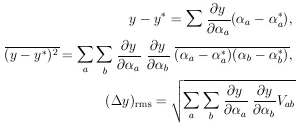(12)

A well-known special case of Eq. (12), which holds only when the variables are completely uncorrelated, is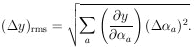In the example of orbit radius in terms ofand v this becomes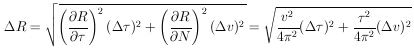in the case of uncorrelated errors. However, ifis non-zero as one might expect, then Eq. (12) gives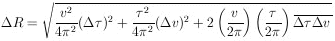It is a common problem to be interested in M physical parameters, y1, ..., yM, which are known functions of thei. In fact the yi can be thought of as a new set ofi or a change of basis fromi to yi. If the error matrix of thei is known, then we have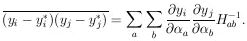(13)

In some such cases the ðyi / ða cannot be obtained directly, but the ði / ðya are easily obtainable. Then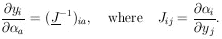Example 3

Suppose one wishes to use radius and acceleration to specify the circular orbit of an electron in a uniform magnetic field; i.e., y1 = r and y2 = a. Suppose the original measured quantities are1 == (10 ± 1)µs and2 = v = (100 ± 2) km/s. Also since the velocity measurement depended on the time measurement, there was a correlated error= 1.5 × 10-3 m. Find r,r, a,a.

Since r = v/ 2= 0.159 m and a = 2v /= 6.28 × 1010 m/s2 we have y1 =12 / 2and y2 = 22 /1. Then ðy1 / ð1 =2 / 2, ðy1 / ð2 =1 / 2, ðy2 / ð1 = -22 /12, ðy2 / ð2 = 2/1 . The measurement errors specify the error matrix asEq. 13 gives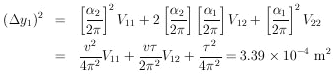Thus r = (0.159 ± 0.184) m

For y2, Eq. 13 gives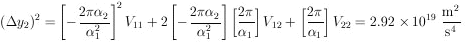Thus a = (6.28 ± 0.54) × 1010 m/s2.